Courses

# Dry Friction Mechanical Engineering Notes | EduRev

## Mechanical Engineering : Dry Friction Mechanical Engineering Notes | EduRev

The document Dry Friction Mechanical Engineering Notes | EduRev is a part of the Mechanical Engineering Course Engineering Mechanics - Notes, Videos, MCQs & PPTs.
All you need of Mechanical Engineering at this link: Mechanical Engineering

Dry Friction

Friction force: When two surfaces come into contact, forces are applied by each surface on the other. The part which is tangent to the contacting surfaces is called the frictional force. If the two surfaces in contact do not move relative to each other, one has static friction. If the two contacting surfaces are moving relative to each other, then one has kinetic (dynamic or slipping) friction.

Static Friction: The frictional force F that can result between two surfaces without having the surfaces slide relative to each other is bounded by the equation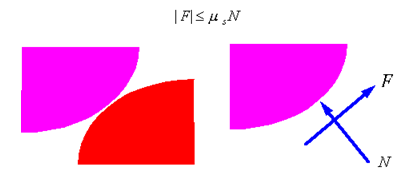whereN is the normal force to the surface, and μsis the static coefficient of friction.

Kinetic friction: The frictional force F that results when two surfaces are sliding on each other is proportional to the normal force applied on the surfaces and is directed opposite to the relative motion of the surfaces. The factor of proportionality is the coefficient of kinetic friction μk,and the equation for F is

F=μ kN

In general the coefficient of kinetic friction is smaller than the coefficient of static friction, which explains the initial difficulty of getting an object to slide.

Pending motion: Pending motion refers to the state just before surfaces start to slip. In this  case  the static frictional force has reached its upper limit and is given by the equation  F=μsN. The direction of the frictional force is opposite to the pending relative motion of the surfaces.

Tipping: When a object starts to tip, it starts to pivot around a point. As a result the contact forces (frictional and normal) must be applied at the pivot point. If the object tips before it slips, then one can find the frictional force from the equations of equilibrium.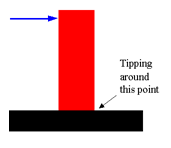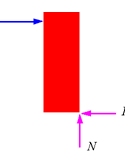Calculating the location of the normal force: If the contact is a surface as opposed to a point, then there is a distribution of normal and frictional forces on that surface. The location of the resultant normal force can be determined from the equilibrium equations.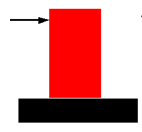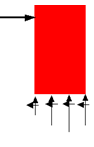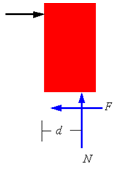Offer running on EduRev: Apply code STAYHOME200 to get INR 200 off on our premium plan EduRev Infinity!

,

,

,

,

,

,

,

,

,

,

,

,

,

,

,

,

,

,

,

,

,

;